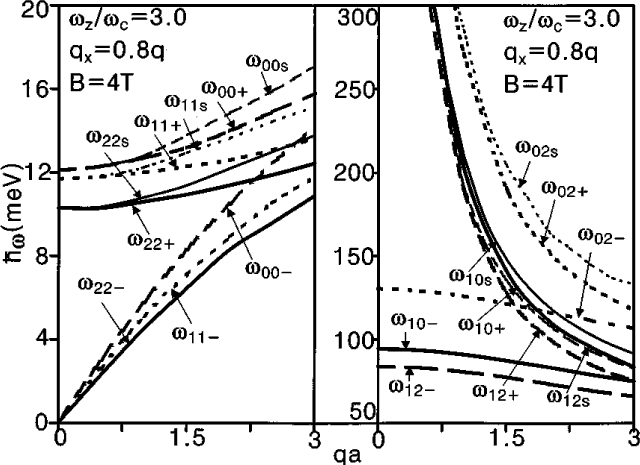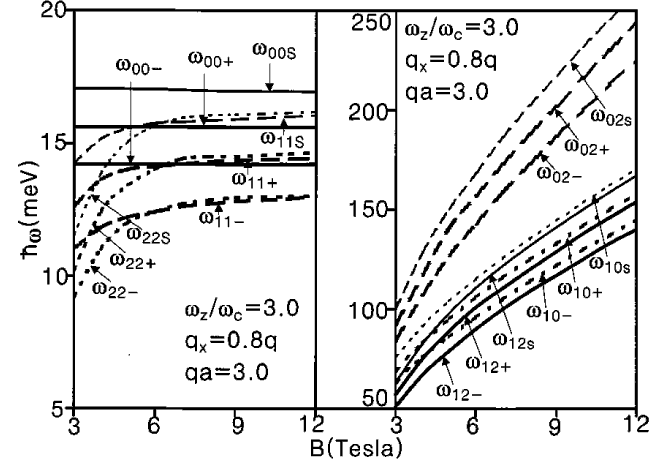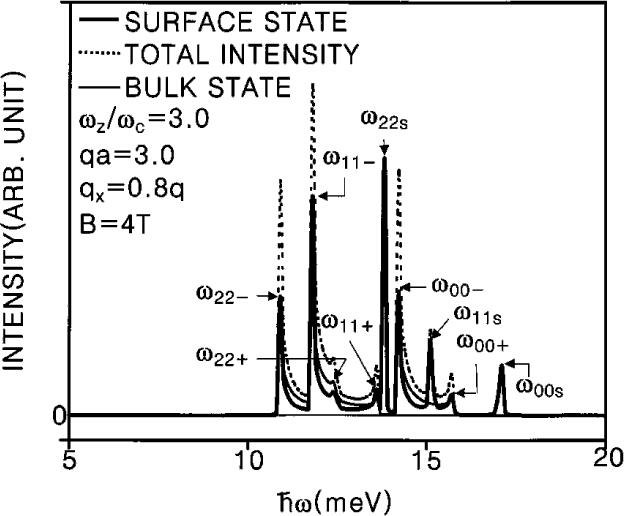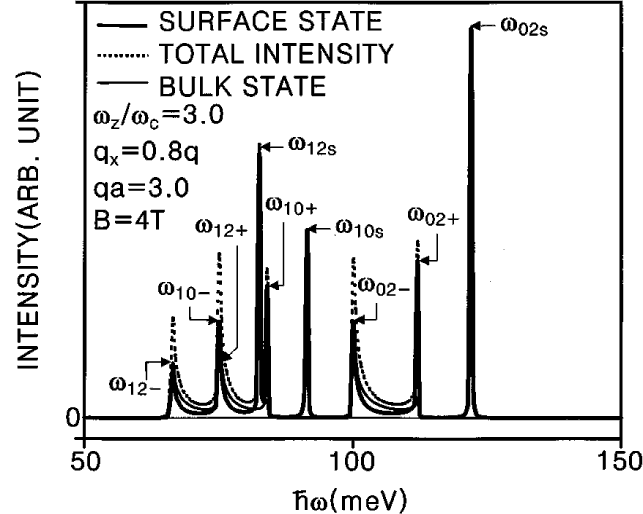Optical properties and collective excitations of plasmons in semiconductor superlattices in magnetic fields
Haeng Ki Lee, Eun Yong Kim, Ki Soo Sohn,Jai Yon Ryu, Suck Whan Kim
Physical Review B (Condensed Matter and Materials Physics) -- 15 January, 2001 -- Volume 63, 045307 (9 pages)
AbstractDispersion relation \ w  versus q a for surface and bulk magnetoplasmons due to the intra- and inter-Landau levels (left-hand side and right-hand side), where the  wij+  and  wij-  are maximum and minimum dispersion energy of the bulk magnetoplasmon modes, respectively, and the   wijS   is the dispersion energy of the surface magnetoplasmon modes. Here i and j represent the Landau level index, respectively. The values of the parameters are as follows: n  = 7.7x1011 cm-2,  a = 82.7 nm, L = 30 nm, m= 0.0665 m0 , e = 13.1, e0 = 1.0, and  Eij = (i-j)hw .Dispersion relation  hw  versus the strength of magnetic field  B  for surface and bulk magnetoplasmons due to the intra- and inter-Landau levels (left-hand side and right-hand side). Here i and j represent the Landau level index, respectively. The values of the parameters are as follows: n  = 7.7x1011 cm-2,  a = 82.7 nm, L = 30 nm, m= 0.0665 m0 , e = 13.1, e0 = 1.0, and  Eij = (i-j)hw .Dispersion relation  hw  versus the ratio of the confining potential parameter to cyclotron resonance frequency  wz/wc  for surface and bulk magnetoplasmons due to the intra- and inter-Landau levels (left-hand side and right-hand side). The values of the parameters are as follows: n  = 7.7x1011 cm-2,  a = 82.7 nm, L = 30 nm, m= 0.0665 m0 , e = 13.1, e0 = 1.0, and  Eij = (i-j)hw .Raman intensities for surface and bulk magnetoplasmons due to the inter-Landau levels at a specific value of qa = 3.0 and kz = 2pa/5. The values of the parameters are as follows: l = a2d = L, and N = 40 and  g  = 0.02 meV.